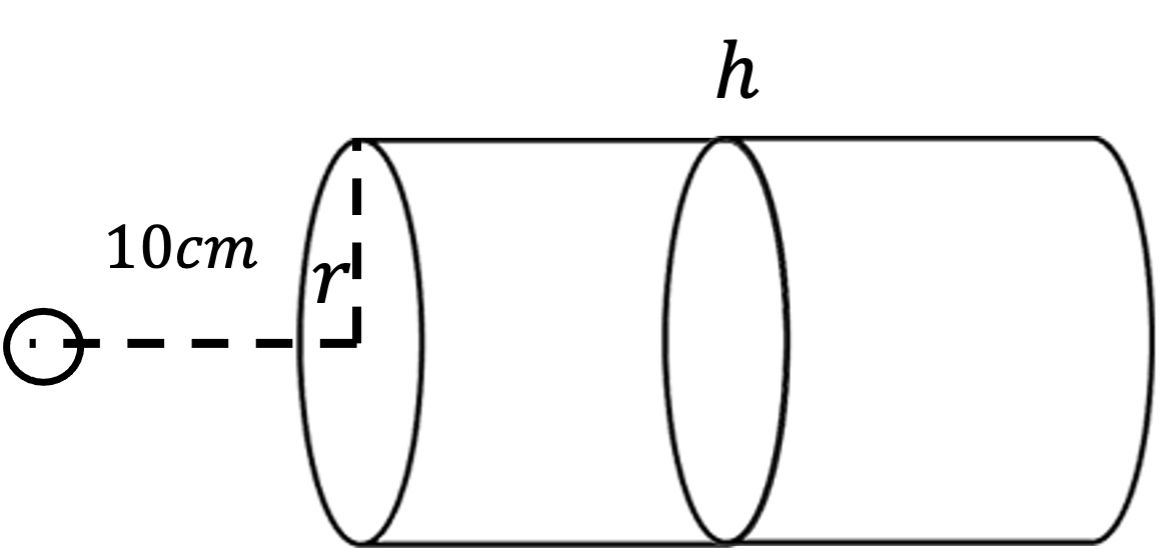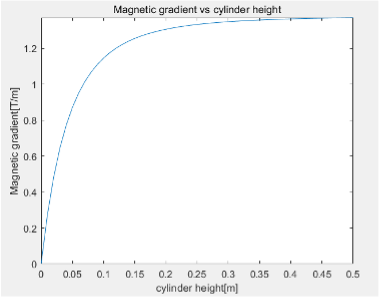Magnetic force: How to choose the right magnet for your design?
02/14/2022 Sijie Ran

From door-catch to electromagnetic crane, many devices used in everyday life rely on magnetic attracting forces to operate. For different applications, it is important to choose the right magnet to achieve the required force. Ideally, we’d like to use the smallest possible magnet to achieve the highest force. However, it is not obvious how we choose the right size magnet for the design. For example, does the bigger magnet always provide bigger force?

For a start, let us introduce the magnetic forces. the magnets exert forces and torques on each other due to the rules of electromagnetism. The most elementary force between magnets is the magnetic dipole-dipole interaction. If all the magnetic dipoles that make up two magnets are known, then the net force on both magnets can be determined by summing up all these interactions between the dipoles.

There are a few different parameters one needs to consider in the magnetic force design. A simple magnet design example, in this case, a cylindrical permanent magnet is attracting a small permanent magnetic sphere from a fixed distance. And we want to select the cylinder magnet so that it can provide the maximum force on the sphere.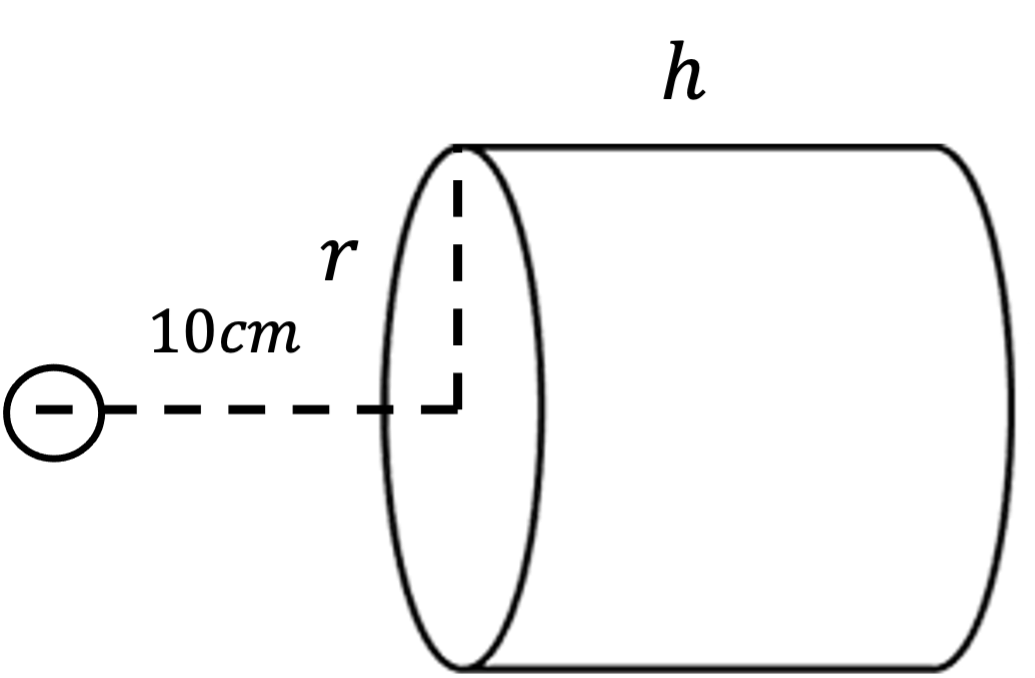The magnetic force between the two magnets can be estimated with the following equation: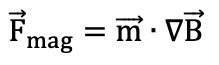One may notice that the magnetic force is proportional to two parameters: The effective magnetic dipole moment of the magnetic sphere, and the magnetic field gradient generated by the bigmagnetic cylinder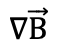. Here we find out an interesting phenomenon. The magnetic force is not really related to how big the magnetic field is, but how fast the magnetic field decays at the sphere’s position (magnetic gradient).

So how do we increase the magnetic gradient so that we can increase the force? Well, one intuitive way of thinking is to increase the size of the cylinder. The bigger the magnet, the higher the gradient it can provide right? Let’s see if we keep the height of the cylinder unchanged and only change the radius of the cylinder, how will the force change?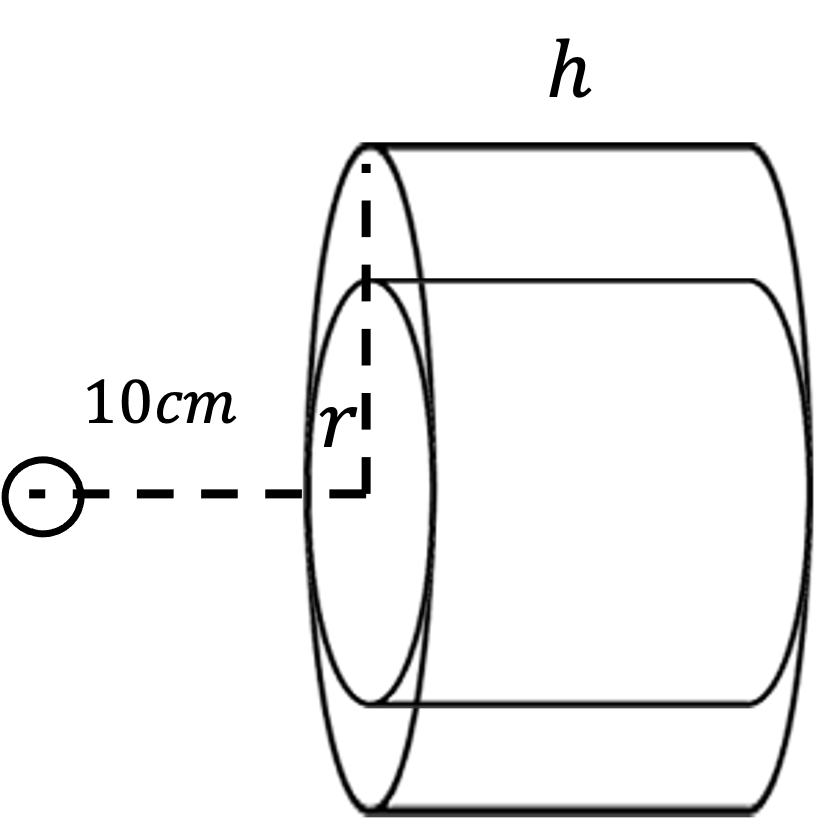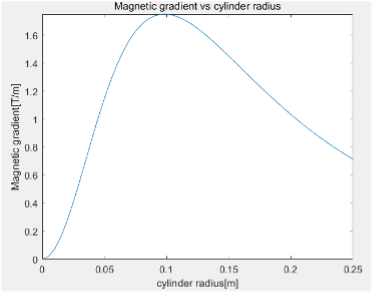We find some interesting observations: at first, when the radius starts to increase, the magnetic gradient magnitude increased as well. However, after passing a certain radius, continuing to increase the radius of the cylinder can no longer increase the magnetic force, on the contrary, the force reduced. The reason behind is that when the surface area becomes too large the extra volume of the magnet actually providing more magnetic field at the sphere local, effectively reducing the magnetic field decay thus reducing the gradient. For a maximum magnetic force on the object, the optimal magnetic surface should have the radius roughly equal to the distance of attracting object. Of course, due to different design configurations it can be changed, but this optimal surface length can be the starting point for your magnet design.

Now we know the optimal surface area for the maximum attracting force. How about increasing the height of our cylinder? Well, increasing the height always increases the force. However, as the height continues to increase, the force increase actually attenuated and eventually will stop. From a practical point of view. As the height of the magnet larger than 3 times of the attracting distance, the increase of height will no longer help with increasing force.